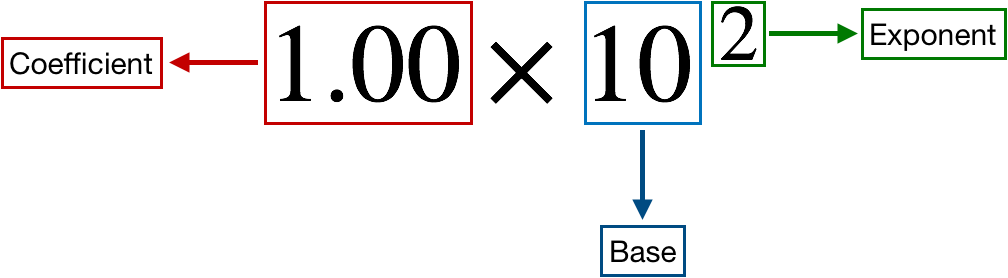# Problem: Express each of the following numbers in exponential notation with correct significant figures:(b) 0.03344

###### FREE Expert Solution

For this problem, we need to express 0.03344 in exponential notation with correct significant figures

Recall that if the number has a decimal point move from left to right. Start counting once you get to your first non-zero number and keep counting until you get to the end.

0.03344 4 SFs

Recall that a scientific notation expression is composed of three parts:81% (439 ratings)###### Problem Details

Express each of the following numbers in exponential notation with correct significant figures:

(b) 0.03344

Frequently Asked Questions

What scientific concept do you need to know in order to solve this problem?

Our tutors have indicated that to solve this problem you will need to apply the Significant Figures concept. You can view video lessons to learn Significant Figures. Or if you need more Significant Figures practice, you can also practice Significant Figures practice problems.

What professor is this problem relevant for?

Based on our data, we think this problem is relevant for Professor Eisenberg's class at BROOKLYN CUNY.

What textbook is this problem found in?

Our data indicates that this problem or a close variation was asked in Chemistry - OpenStax 2015th Edition. You can also practice Chemistry - OpenStax 2015th Edition practice problems.